Understanding mean Average Precision for Object Detection (with Python Code)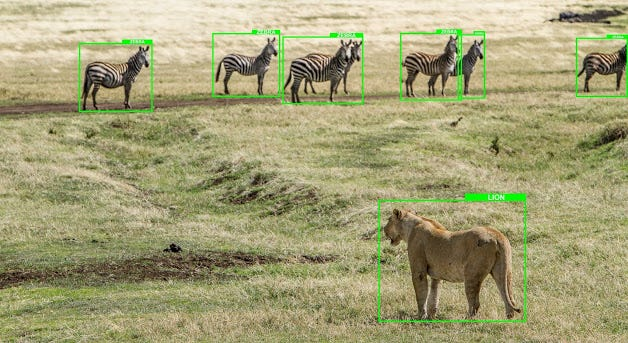Photo by Avel Chuklanov on Unsplash

If you ever worked on object detection problem where you need to predict the bounding box coordinates of the objects, you may have come across the term mAP (mean average precision). mAP is a metric used for evaluating object detectors. As the name suggest it is the average of the AP.

To understand mAP , first we need to understand what is precision, recall and IoU(Intersection over union). Almost everyone is familiar with first two terms, in case you don’t know these terms I am here to help you.

Precision and Recall

Precision: It tells us how accurate is our predictions or proportion of data points that our model says relevant are actually relevant.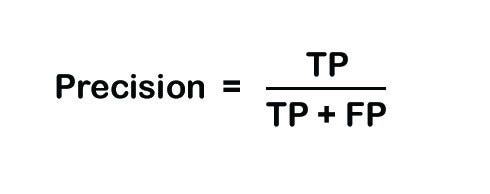Formula for precision

Recall: It is ability of a model to find all the data points of interest or relevant cases. In other words it is the measure of how good our model find out all the positives.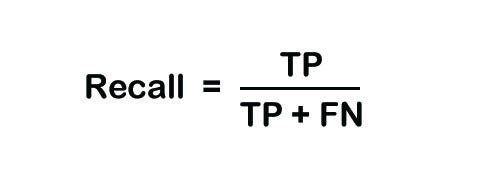Formula for recall

One thing to Note here is that, If we increase precision, recall will decrease and vise versa.

If you want to learn precision and recall more deeply then go through this article where I explained precision and recall with example.

Now, let’s move to our next term that is IoU (Intersection over union).

IoU(Intersection over union)

In simple words, IoU is the ratio of the area of intersection and area of union of the ground truth and predicted bounding boxes. Here, “ground truth bounding box” refers to the actual bounding box whose coordinates are given in the training set. Let’s understand it with the help of an image.

In the above image, the green box is the actual box and the red box is the box that our model predicted as shown in the image. I know that object detection models can detect this Doraemon toy more accurately but for shake of this example let us assume that our model detected it as shown above.

Now it can be clearly seen that the actual and predicted bounding boxes have different coordinates. Area of intersection is the common area covered by both bounding boxes or the area where one box overlaps the other box and area of union is the total area covered by both the bounding boxes. So the formula for IoU is: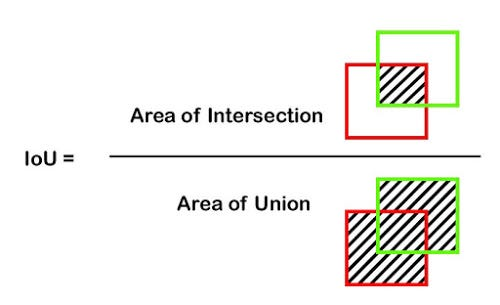Formula for IoU

Now you might have a question that why we are calculating this IoU in the first place and how it is going to help us with calculating mAP ?, Answer is, IoU helps us in determining whether a predicted box is a true positive, false positive or false negative. we predefine a threshold value for IoU say 0.5 which is commonly used.

• If IoU > 0.5 then it is a true positive,
• if IoU< 0.5 it is a false positive and,
• if IoU > 0.5 but object is miss classified then it will be a false negative.

One thing to note here is that there is no “True negative” because it is assumed that the bounding box will always have something inside it, which means a bounding box will never be empty and hence there will be no true negative.

Now that we know what is precision, recall and IoU, its time to start calculating mAP. To calculate mAP we first have to calculate Precision, Recall and IoU for each object.

Working on a dataset

For this article I created two small custom datasets using 10 images. One for holding the actual coordinates and the other for holding the predicted coordinates. Then I merged the predicted coordinates with the original dataframe and came up with a final dataframe which holds image names, object class, actual bounding box coordinates and the predicted bounding box coordinates. By coordinates I mean the xmin, ymin, xmax and ymax. You can assume this dataset as a validation set for object detection.

So let’s dive into the python code. Starting with importing libraries and data.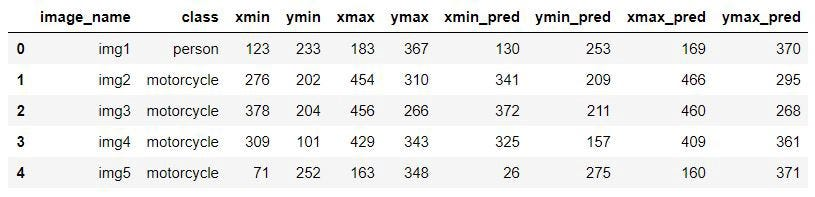Dataframe

Now, we will create a function to calculate IoU. We will pass a dataframe to this function and it will return IoU values.

Next, we will call IoU function using apply function to apply over each row of the dataframe. But before that, we will create a new dataframe for our metric table.

So now we have got out IoU values, we can move towards finding out whether predicted box is TP, FP or FN. For this we will create a column ‘TP/FP’ which will hold TP for true positive and FP for false positive. we will use IoU threshold as 0.5.

Now, we will calculate precision and recall by iterating over each row of the dataframe.

Now we have Precision, Recall and IoU calculated, there is one thing left to be calculated and then we are good to go for calculating mAP and that thing is IP(Interpolated Precision).

Interpolated Precision: It is simply the highest precision value for a certain recall level. For example if we have same recall value 0.2 for three different precision values 0.87, 0.76 and 0.68 then interpolated precision for all three recall values will be the highest among these three values that is 0.87.Formula for Interpolated Precision

Now let’s calculate IP.

This is how our final dataframe looks like.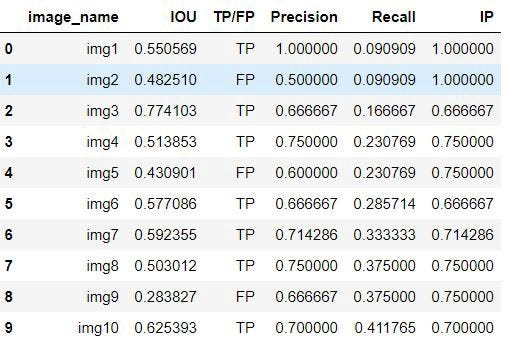Final Dataframe

Finally, It’s time to calculate mAP. To calculate mAP we will take the sum of the interpolated precision at 11 different recall levels starting from 0 to 1(like 0.0, 0.1, 0.2, …..).Average Precision at 11 recall levels

We will first create an empty list to store precision value at each recall level and then run a for loop for 11 recall levels.

This is it, we have calculated our mAP for object detection. Please note that this is not the only way to calculate mAP. This is how I calculated it. Also for the simplicity of the code, I didn’t include the false negative cases. You can do that by doing some changes in the code.

Watch this video and learn how pooling layer works in CNN.

Check out my other article on neural networks where I explained neural networks as simple as possible.

1.Can you please explain the formula of FN in the above program ..

Thanks,
Karuna Sree

2.Thanks for the blog ... I couldn't understand the formula FN = len(eval_table['TP/FP']== 'TP') .... can you please help me ..

Thanks,
Karuna Sree

1.It is mentioned above that if IOU>0.5 then it will be true positive and also if IOU>0.5 but object is miss classified then it will be flase negative(FN). So FN is the number of count for IOU>0.5 which is TP.

2.Thank you .. But how are you getting the misclassified ... Not all true positives are not FN right ? Are you comparing that classification difference here ?

3.Yes, you are right that all TP are not FN but finding FN will complicate the code. So, just for simplicity we are considering them equal to TP. You can make it zero or you can replace some TP with FN in the data for your calculation and understanding. For better understanding I will mention this assumption in the article.